# Improvement of Free Convection Heat Transfer in a Concentric Cylindrical Annulus Heat Exchanger Using Nanofluid

Improvement of Free Convection Heat Transfer in a Concentric Cylindrical Annulus Heat Exchanger Using Nanofluid
Laboratory of Pure and Applied Mathematics “LMPA”, Department of Mathematics, Faculty of Mathematics and Informatics, M. Boudiaf M’sila University, 28000, Algeria

Faculty of Sciences and Technology, Mohamed El Bachir El Ibrahimi University, Bordj Bou Arreridj, El-Anasser, 34030, Algeria

Energy Physics Laboratory, Department of Physics, Faculty of Science, Frères Mentouri Constantine1 University, 25000, Algeria

Corresponding Author Email:
t.tayebi@univ-bba.dz
Page:
566-574
|
DOI:
https://doi.org/10.18280/mmep.060412
31 January 2019
|
Accepted:
7 December 2019
|
Published:
24 December 2019
| Citation

OPEN ACCESS

Abstract:

A numerical study based on the analysis of laminar natural convection in a concentric cylindrical annulus heat exchanger is investigated. The operating fluid is confined between two horizontal concentric cylinders which are maintained at different uniform temperatures. The governing equations the flow (of continuity, momentum and energy) are numerically solved via finite volume method (FVM). The investigation is performed for Rayleigh number and volume fraction of nanoparticles in the range of 103-105 and 0-12%, respectively. The effective thermal conductivity and viscosity of the nanofluids mixture are calculated via Maxwell-Garnett model (MG-model) and Brinkman model, respectively. The results are presented in terms of isotherms, fluid flow patterns and Nusselt number distribution function of Rayleigh number and the volume fraction of silver nanoparticles. The results are also discussed in detail. Results are discussed in detail. It is found that a very good agreement exists between the present results and those from the literature. It is found that fluid flow intensity and heat transfer rate increase with the increase of the nanoparticles volume fraction and Rayleigh number, Also, the thermal effectiveness depends on the Rayleigh number and the volume fraction of the nanoparticles.

Keywords:

nanofluids, natural convection, horizontal concentric cylinders, finite volume method

1. Introduction

Natural convection in enclosures has been the subject of several studies which justifies its involvement in many industrial applications such as heat exchangers, furnaces, solar collectors, building design, cooling of electronic components, etc. The references of these studies can be classified according to their character: experimental [1-3], theoretical [4, 5] or numerical [3, 6-34]. In this last case, solutions are in the steady [6-31] or unsteady state [32-34] and the space is two-dimensional [6-19, 23-34] or tridimensional [20-22, 25]. The enclosures have various geometries, rectangular or cubical [6-21, 32, 34], cylindrical , spherical  or also in the form of cylindrical annulus [23-26] and elliptical [27-30].

The need to improve the heat transfers in such applications has given birth to the development of nanofluids which are colloidal solutions containing nanoparticles in suspension with a base fluid. For more information, readers are referred to the work of Menni et al. , who presented a review of numerical studies on the modeling of convection heat transfer in nanofluids in the complex geometries.

The annular cavity, in particular, is often used as an exchanger in energy conversion. Examples of applications include industrial water heating and medical sterilization. In the following, a literature review of the principal investigations is carried out that focused on the convection in annular passages using the nanofluids as a working fluid and thus it’s related to our study. Sheikholeslami and Chamkha  have examined natural convection heat transfer of a Fe3O4–ethylene glycol nanofluid in a semi-annulus enclosure with a sinusoidal wall in the presence of an electric field. Dawood el al.  conducted a numerical study for laminar mixed convection heat transfer flow for different types of nanofluids (Al2O3–water, CuO-water, SiO2-water, and ZnO-water) in an 3D elliptic annular passage with constant heat flux. The numerical study was carried out via the finite volume method (FVM) using of the SIMPLE algorithm. They mentioned that SiO2–water nanofluid has the highest heat transfer rate followed by Al2O3-water, ZnO-water, CuO-water, and pure water, in this order. The heat transfer rate in the annulus increased by increasing both nanoparticle concentration and Reynolds number, and decreased by increasing the nanoparticle size. A numerical study of steady-state laminar natural convective heat transfer and fluid flow in an annulus between two horizontal eccentric cylinders filled with copper-water nanofluid is presented by Tayebi et al. . They reported that the addition of Cu nanoparticles affected the temperature gradient around the hot inner cylinder wall except in the plume region for high values of Rayleigh number. In addition, the mean Nusselt number and the intensity of the flow increase by increasing the nanoparticles concentration. Tayebi and Chamkha [24, 28]. numerically investigated heat transfer by natural convection in an annular space between two eccentric horizontal cylinders  and confocal elliptic cylinders  ﬁlled with water-based hybrid nanoﬂuid. The inner cylinder is heated isothermally while the outer one is cooled. They noticed that the use of Cu-Al2O3/water hybrid nanoﬂuids granted higher heat transfer rates compared to the Al2O3/water nanoﬂuids with the same volume fractions. Dogonchi et al.  have examined numerically MHD natural convection heat transfer of Cu-water nanofluid in a porous region between hot inner rectangular cylinder and cold outer circular cylinder. Natural convection and Cu-water nanofluid flow in an annular region among confocal elliptic cylinders was examined by Tayebi et al. . In another study , they have explored free convection in an annulus between confocal elliptical cylinders using CNT-water nanofluid.

This paper is an additional contribution to the study of the impact of pertinent parameters on natural convection in a concentric cylindrical annulus heat exchanger filled with a nanofluid. The finite volume method is chosen to simulate this problem. Effect of nanoparticles volume fraction, Rayleigh number on heat transfer and fluid flow are examined in detail and illustrated graphically. This present configuration can be seen in many practical industrial and engineering applications, such as in solar thermal collector’s design, thermal building design, air conditioning, chemical processing equipment, drying technology, lubrication, etc.

2. Mathematical Formulation

2.1 Problem description

The geometry adopted in this study is an annular formed by two horizontals and coaxial (concentric) cylinders with a radii ratio C=R2/R1=2 filled with Ag-water based nanofluid. The inner and the outer cylindrical walls are maintained isotherm at the temperature Tc and Tf, respectively with Tc> Tf. The base ﬂuid (water) and the solid spherical nanoparticles (Silver) are in thermal equilibrium. The thermophysical properties of the nanoﬂuid are considered constant with the exception of the density which varies according to the Boussinesq approximation. The viscous dissipation in the heat equation and the work of the pressure forces are negligible, the radiation is not considered (the emissive properties of the two walls of the cylinder are neglected). We assume that the problem is two-dimensional, steady-state and laminar. The schematic view of the annulus right section is shown in Figure 1.

The thermo-physical properties of the base fluid and different types of nanoparticles used for code validation are given in Table 1.

## 1.jpgFigure 1. Geometry with boundary conditions

Table 1. Thermophysical properties of the water and Silver nanoparticles 

 $\rho$ (kg.m-3) Cp (J.kg1K1) K (W.m-1 K-1) $\beta$ (K-1) Pure Water 997.1 4179 0.613 21 × 10-5 Silver 10500 235 429 1.89 × 10-5

2.2 Governing equations

The equations of the 2D laminar steady natural convection under Boussinesq approximation, are written as following:

• Continuity equation:

$\frac{\partial U}{\partial x}+\frac{\partial V}{\partial y}=0$   (1)

• Momentum equations:

$U \frac{\partial U}{\partial x}+V \frac{\partial U}{\partial y}=-\frac{1}{\rho_{n f}} \frac{\partial P}{\partial x}+v_{n f}\left(\frac{\partial^{2} U}{\partial x^{2}}+\frac{\partial^{2} U}{\partial y^{2}}\right)$   (2a)

$U \frac{\partial V}{\partial x}+V \frac{\partial V}{\partial y}=-\frac{1}{\rho_{n f}} \frac{\partial P}{\partial y}+$$\frac{(\rho \beta)_{n f}}{\rho_{n f}}\left(T-T_{c}\right)+v_{n f}\left(\frac{\partial^{2} V}{\partial x^{2}}+\frac{\partial^{2} V}{\partial y^{2}}\right) (2b) • Energy equation: U \frac{\partial T}{\partial x}+V \frac{\partial T}{\partial y}=(\alpha)_{n f}\left(\frac{\partial^{2} T}{\partial x^{2}}+\frac{\partial^{2} T}{\partial y^{2}}\right) (3) • Vorticity equation: U \frac{\partial \omega}{\partial x}+V \frac{\partial \omega}{\partial y}=g \frac{(\rho \beta)_{n f}}{\rho_{n f}}\left(\frac{\partial T}{\partial x}\right)+v_{n f}\left(\frac{\partial^{2} \omega}{\partial x^{2}}+\frac{\partial^{2} \omega}{\partial y^{2}}\right) (4) with: \omega=\frac{\partial V}{\partial x}-\frac{\partial U}{\partial y} (5) By introducing the current function \psi such that: \left\{\begin{array}{l}{U=\frac{\partial \psi}{\partial y}} \\ {V=-\frac{\partial \psi}{\partial x}}\end{array}\right. (6) We deduce: \omega=-\left(\frac{\partial^{2} \psi}{\partial x^{2}}+\frac{\partial^{2} \psi}{\partial y^{2}}\right) (7) The adimensionalisation has the advantage of facilitating the simplification of the equations and generalizing the results. By making the following dimensionless quantities: X^{*}=\frac{x}{\left(R_{2}-R_{1}\right)} ; \quad Y^{*}=\frac{y}{\left(R_{2}-R_{1}\right)} ; \quad U^{*}=\frac{U\left(R_{2}-R_{1}\right)}{\alpha_{f}} ; \quad V^{*}$$=\frac{V\left(R_{2}-R_{1}\right)}{\alpha_{f}}$

$P^{*}=\frac{p\left(R_{2}-R_{1}\right)^{2}}{\rho_{f} \alpha_{f}^{2}} ; T^{*}=\frac{\left(T-T_{f}\right)}{\left(T_{c}-T_{f}\right)} ; \psi^{*}=\frac{\psi}{\alpha_{f}} \cdot \omega^{*}=\omega \frac{\left(R_{2}-R_{1}\right)^{2}}{\alpha_{f}}$

The above equations are written in the dimensionless form as:

$\frac{\partial U^{*}}{\partial X^{*}}+\frac{\partial V^{*}}{\partial Y^{*}}=0$ (8)

$U^{*} \frac{\partial U^{*}}{\partial X^{*}}+V^{*} \frac{\partial U^{*}}{\partial Y^{*}}=-\frac{\rho_{f}}{\rho_{n f}} \frac{\partial P^{*}}{\partial X^{*}}+\operatorname{Pr} \frac{v_{n f}}{v_{f}}\left(\frac{\partial^{2} U^{*}}{\partial X^{* 2}}+\frac{\partial^{2} U^{*}}{\partial Y^{* 2}}\right)$ (9)

$U^{*} \frac{\partial V^{*}}{\partial X^{*}}+V^{*} \frac{\partial V^{*}}{\partial Y^{*}}=-\frac{\rho_{f}}{\rho_{n f}} \frac{\partial P^{*}}{\partial Y^{*}}+\operatorname{Pr} \frac{v_{n f}}{v_{f}}\left(\frac{\partial^{2} V^{*}}{\partial X^{* 2}}+\frac{\partial^{2} V^{*}}{\partial Y^{* 2}}\right)+$$G r P r^{2} \frac{(1-\varphi) \rho_{f} \beta_{f}+\varphi \rho_{s} \beta_{s}}{\rho_{n f} \beta_{f}} T^{*}$ (10)

$U^{*} \frac{\partial T^{*}}{\partial X^{*}}+V^{*} \frac{\partial T^{*}}{\partial Y^{*}}=\frac{\alpha_{n f}}{\alpha_{f}}\left(\frac{\partial^{2} T^{*}}{\partial X^{* 2}}+\frac{\partial^{2} T^{*}}{\partial Y^{* 2}}\right)$ (11)

$\omega^{*}=-\left(\frac{\partial^{2} \psi^{*}}{\partial X^{* 2}}+\frac{\partial^{2} \psi^{*}}{\partial Y^{* 2}}\right)$ (12)

Pr and Gr are the following dimensionless numbers:

$\operatorname{Pr}=\frac{v_{f}}{\alpha_{f}}, \quad G r=\frac{g \beta_{f}\left(R_{2}-R_{1}\right)^{3}\left(T_{H}-T_{c}\right)}{v_{f}^{2}}$ and $\quad R a=G r \times P r$

The effective density of the nanofluid is:

$(\rho)_{n f}=\varphi \rho_{p}+(1-\varphi) \rho_{f}$ (13)

Thermal diffusivity of the nanofluid is:

$(\alpha)_{n f}=\frac{K_{n f}}{\left(\rho c_{p}\right)_{n f}}$ (14)

The heat capacitance of the nanofluid given as:

$\left(\rho C_{p}\right)_{n f}=\varphi\left(\rho C_{p}\right)_{p}+(1-\varphi)\left(\rho C_{p}\right)_{f}$ (15)

The thermal expansion coefficient of the nanofluid can be determined by:

$(\rho \beta)_{n f}=\varphi(\rho \beta)_{p}+(1-\varphi)(\rho \beta)_{f}$ (16)

The effective dynamic viscosity of the nanofluid given by Brinkman  is:

$(\mu)_{n f}=\frac{\mu_{f}}{(1-\varphi)^{2.5}}$ (17)

In Eq. (14), Knf is the effective thermal conductivity of the nanofluid, which for low dense mixtures with micro-sized spherical particles, according to Maxwell , is:

$K_{n f}=K_{f} \frac{\left(K_{p}+2 K_{f}\right)-2 \varphi\left(K_{f}-K_{p}\right)}{\left(K_{p}+2 K_{f}\right)+\varphi\left(K_{f}-K_{p}\right)}$ (18)

• Boundary conditions formulation

The corresponding boundary conditions take the following form:

At the both cylinders surface:

$U^{*}=V^{*}=\frac{\partial^{2} \psi^{*}}{\partial X^{* 2}}=\frac{\partial^{2} \psi^{*}}{\partial Y^{* 2}}=0$ (19)

$\omega^{*}=-\left(\frac{\partial^{2} \psi^{*}}{\partial X^{* 2}}+\frac{\partial^{2} \psi^{*}}{\partial Y^{* 2}}\right)$ (20)

$T^{*}$=0, at the outer boundary

$T^{*}$=1, at the inner boundary

2.3 Evaluation of Nusselt number

The local Nusselt number along the heat inner and outer cylinders based on the hydraulic diameter of the annulus and by using the definition of the dimensionless temperatures is evaluated from the following relation:

$N u_{l o c}=\frac{-K_{n f}}{K_{f}}\left(\frac{\partial T^{*}}{\partial n}\right)$ (21)

The mean Nusselt number is expressed from the following relation:

$N u_{A v g}=\frac{\sum_{n œ u d} N u_{l o c}}{\text { number of nodes }}$ (22)

3. Numerical Procedure and Validation

The equations governing the convective flow are solved via the ﬁnite volume method based on the SIMPLE algorithm. The standard discretization scheme is used for the pressure equation of and the second-order upwind scheme is performed for momentum and energy equations by using under relaxation coefficients in order to control the convergence of the solution. To verify the effect of the mesh on the numerical results, several uniforms meshes have been used arbitrarily. In Table 2 we present the mean Nusselt number values and the maximum values of stream function depending on the number of nodes for two Rayleigh number cases 104 and 105 using the pure water. the results allowed us to choose the mesh with 13200 nodes.

Table 2. Study of the mesh effect

 Number of nodes 900 3400 13200 32000 Ra=103 $\overline{N u}$ 2.869 2.836 2.803 2.798 $\psi^{*} \max$ 0.537 0.495 0.484 0.481 Ra=104 $\overline{N u}$ 5.56 5.2 5.09 5.08 $\psi^{*} \max$ 3.086 2.89 2.48 2.477

## 2.jpgFigure 2. Comparison between our work results and those of Abu-Nada et al. , Tayebi et al.  et Kuhen and Goldstein 

For a natural convection flow between two cylinders where the annulus is filled with water, there are several numerical and experimental studies, which have been used in this work to check our numerical results. In this fact, our numerical results are compared with the numerical study of Abu-Nad et al. , Tayebi et al.  and experimental works of Kuhen and Goldestein . These works are carried out for a Rayleigh number Ra=47000 and a radii ratio C=R2/R1=2.6. We observe that, for the four results, the change in temperature is comparable.

4. Results and Discussion

The numerical simulation treats the problem of natural convection nanofluid flow in an annular space delimited by two horizontal cylinders and filled with silver-water nanofluid. We consider two horizontal coaxial cylinders characterized by a radii ratio C=2, the outer cylindrical wall is maintained isotherm under a cold temperature Tf and the inner cylindrical wall is maintained isotherm under a temperature Tc (Tc>Tf). The simulation is done for a range of the Rayleigh number of 103 to 105 and the following values of the volume fraction of the nanoparticles:  $\varphi$= 0, 0.03, 0.06, 0.09 and 0.12.

## 3.pngFigure 3. Average Nusselt number on the hot wall versus $\varphi$ for different Rayleigh number

## 4.pngFigure 4. Average Nusselt number on the hot wall versus Rayleigh number for different concentrations

Figure 3 shows the evolution of average Nusselt number along the inner cylinder in function of the volume fraction of silver nanoparticles at different values of Rayleigh number. According to this figure, we observe that the average Nusselt increases with the increase of the volume fraction for all Rayleigh numbers. Also, for a given concentration, $\varphi$, the mean Nusselt values for Ra=105 are always greater than those of 103 and 104. The variation of average Nusselt, at the hot wall according to Rayleigh number for different nanoparticles concentrations of silver, is shown in Figure 4. It’s clear that for all the values of the volume concentration, the average Nusselt number increases with the increase of Rayleigh number. This is because as Rayleigh number and volume fraction increase, the flow intensity increases and the temperature gradients near the active wall increase as well.

## 5a.png(a)

## 5b.png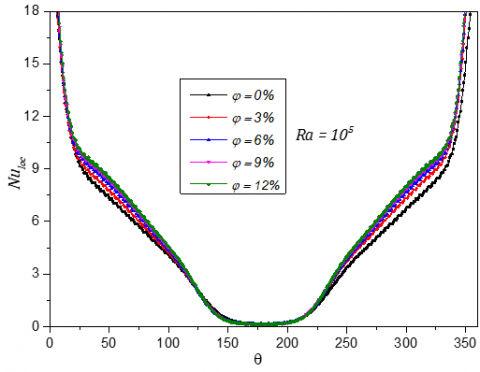(b)

Figure 5. Variation of local Nusselt number along inner wall (a) and outer wall (b) for different concentrations and for Ra=105

The Figure 5 shows the profiles of local Nusselt numbers variation along the inner and the outer walls for different values of $\phi$ at Ra=105. We notice, in these figures, that the mean Nusselt number values on the inner wall are minimal at the plume region that corresponds to the angular position $\theta$=0° (between the two contra-rotating vortices), while taking its maximum values at this region on the outer one.

Figure 6 illustrates the evolution of the maximum stream function with respect to the volume fraction of Ag nanoparticles at different Rayleigh number. As it’s expected, there is a linear relationship between $\psi_{\max }^{*}$ and $\varphi$, if $\varphi$ increases, $\psi_{\max }^{*}$ also increases. For a low value of Rayleigh number of about (103), the heat transfer inside the cavity is mainly done by conduction when the maximum absolute values of the stream function are small and increase slightly with the volume concentration. The heat transfer mechanism becomes dominant by the increase of Rayleigh number and the flow intensity increases considerably by increasing the volume concentration.

## 6.png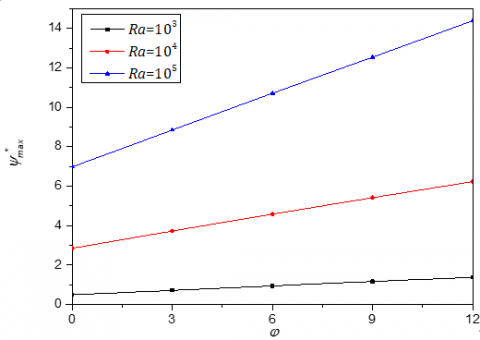Figure 6. Variations of the maximum stream function with respect to the concentrations of different Rayleigh number

The Figures 7 and 8 above show the dimensionless temperature profiles inside the cavity at the position $\theta$=90° in presence of the silver nanoparticles. It’s observed that the maximum values of the temperature are near the inner wall and low values are in the vicinity of the outer one corresponding the thermal boundary conditions. In the case of Ra=103, we see that the distribution of the temperature is a straight line of negative slope corresponding to a purely conductive regime where the fluid behaves like a solid that transmits heat by thermal conduction from the hot wall to the cold wall (Figure 7). For a given value of Rayleigh number: Ra=105, the temperature profiles remain substantially unchanged with respect to the concentration, $\varphi$ (Figure 8).

Figures 9-11 display the streamlines (right side) and the isotherms (left side), for different values of Rayleigh number (Ra=103-105) and for different volume concentrations of silver nanoparticles ($\varphi$=0-12%). These figures show that for all cases, the flow is symmetrical and is organized in two cells. On the left side, the flow rotates in the trigonometric direction and on the right side; it’s in contrary direction (the fluid particles move upward under the action of the gravitational forces and cool on the cold wall).  For low values of Rayleigh number (Ra=103), the isotherms are almost parallel and fit quite well the wall profiles. In this case, the temperature distribution is just decreasing from the hot wall to the cold wall and the values of the streamlines which are given on these figures are very small. We can say that heat transfers are mainly done by conduction. For Ra=104 et 105, the isotherms change substantially and the streamlines values mentioned in the same figures also increase substantially, which translates a transformation from conductive transfer to convective transfer. A plume region appears on the top of the hot inner cylinder (at the angular position $\theta$=0). In addition, by increasing the Rayleigh, the density of the isotherms at the level of the walls increases (temperature gradient increases) and therefore, the mean Nusselt number increases (see Figure 4). Moreover, it’s observable that by increasing the Rayleigh number, the main central vortex se deforms from an oval shape to a stretched form (aerodynamic profile), the inner vortex center moves upward because of the buoyancy effect and the lower half of our space becomes a quasi-static area (inert area). The amplitudes of the streamlines increase by increasing the volume concentration of the silver nanoparticles and this increase becomes more considerable for the high values of the Rayleigh number.

## 7.png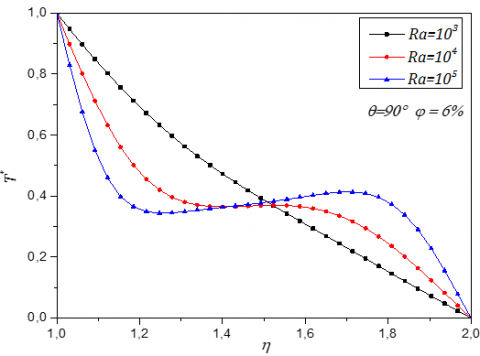Figure 7. Dimensionless temperature Profile along the middle section $\theta$=90° for different Rayleigh number and for $\varphi$=6%

## 8.pngFigure 8. Temperature profile along the middle section $\theta$=90° for different concentrations and for Ra=105

## 9a.jpg## 9b.jpgFigure 9. Isotherms (left) and streamlines (right) for Rayleigh number Ra=103 and for different volume concentrations

## 10a.png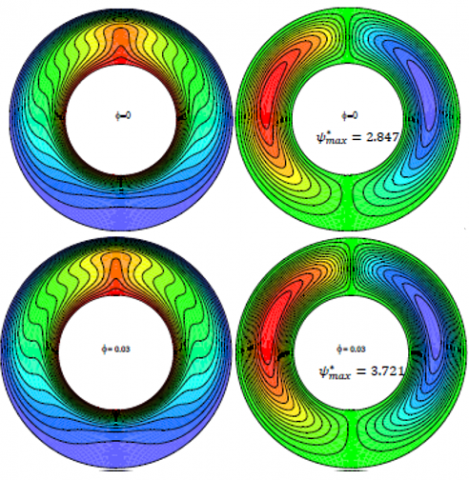## 10b.jpg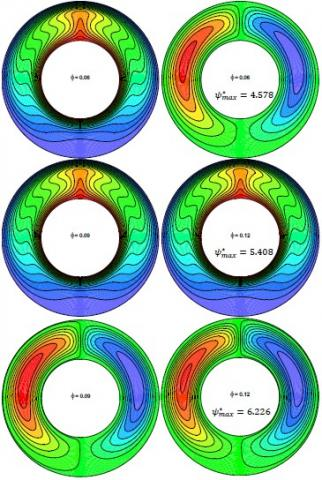Figure 10. Isotherms (left) and streamlines (right) for Ra=104 and different volume concentrations $\varphi$

## 11a.png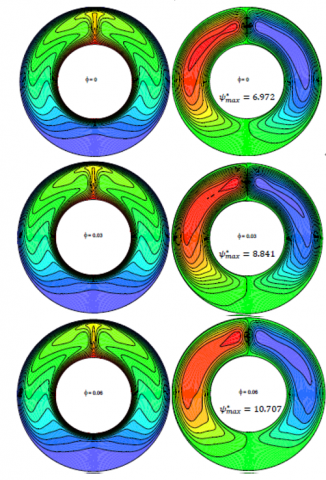## 11.jpgFigure 11. Isotherms (left) and streamlines (right) for Ra=105 and different volume concentration $\varphi$

Table 3. Heat transfer effectiveness

 $\eta$(%) $\varphi$=3% $\varphi$=6% $\varphi$=9% $\varphi$=12% Ra=103 3.385 6.569 8.905 10.269 Ra=104 8.156 13.582 16.847 18.566 Ra=105 7.439 12.624 15.859 17.574

## 12.jpg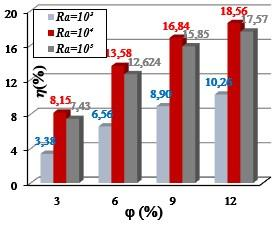Figure 12. Histogram of the heat transfer effectiveness

Table 3 and the histogram of Figure 12 show in percentage terms (%), the effectiveness $\eta$(%) of the heat transfer rate with respect to the pure water for our configuration. It’s clear that the dispersion of the silver nanoparticles with high thermal conductivity within the water increases the effective conductivity of the mixture and also improves the heat transfer inside the annulus. These increases depend on the Rayleigh number where the maximum effectiveness values are recorded for moderate Rayleigh numbers (Ra=104).

The expression of improvement is defined by:

$\eta(\%)=\frac{N u_{A v g}(\varphi)-N u_{A v g}(\varphi=0)}{N u_{\operatorname{moy}}(\varphi=0)} \times 100$

5. Conclusion

The main conclusions are summarized as follows:

• The flow is kept symmetrical inside the annulus with respect to the vertical center line regardless of the Rayleigh number and the volume fraction of nanoparticles.
• As Rayleigh increases, convection amplifies and the effect of volume concentration of nanoparticles on the intensity of flow is greater for high Rayleigh numbers
• Employing nanofluids with Silver nanoparticles increases the rate of heat transfer with the increase of $\varphi$.
• The flow intensity increases with the increase of the nanoparticles volume fraction for the whole range of Rayleigh.
• The thermal effectiveness depends on the Rayleigh number and the volume fraction of the nanoparticles.
• Maximum improvement rate values are obtained at moderate Rayleigh numbers (Ra=104), while, minimum values are recorded for low Rayleigh values.

In conclusion, the present study indicates the need for more investigation to arrive at a complete understanding of physical phenomenon before using these nanofluids in practical applications.

Nomenclature

Cp     Specific heat at constant pressure (J.kg-1.K-1)

Gr     Nombre de Grashof

g       Gravitational acceleration (m.s-2)

H      Side of the enclosure (m)

Nu    Nusselt number

Nuavg Average Nusselt number

P       Pressure (N.m-2)

Pr     Prandtl number

T       Fluid’s temperature (K)

U      Velocity component-coordinate x (m.s-1)

V       Velocity component-coordinate y (m.s-1)

x,y,z  Cartesian coordinates (m)

Greek letters

$\gamma$        Angle of inclination (°)

$\beta$      Thermal expansion coefficient (K-1

$K$      Thermal conductivity (W.m-1.K-1

$v$      Kinematic viscosity (m2.s-1)

$\rho$      Density (kg.m-3)

$\psi$      Stream function (m2.s-1)

$\omega$     Vorticity (s-1)

$\varphi$      Volume fraction of the nanoparticles

Superscripts

*       Dimensionless parameters

Subscripts

C      Cold

H      Hot

f        Fluid (pure water)

p       Solid nanoparticles

nf      Nanofluid

References

 Kuehn, T.H., Goldstein, R.J. (1976). An experimental and theoretical study of natural convection in the annulus between horizontal concentric cylinders. Journal of Fluid Mechanics, 74(4): 695-719. https://doi.org/10.1017/S0022112076002012

 Kuehn, T.H., Goldstein, J. (1978). An experimental study of natural convection heat transfer in concentric and eccentric horizontal cylindrical annuli. ASME J. Heat Transfer, 100(4): 635-640. https://doi.org/10.1115/1.3450869

 Charrier-Mojtabi, M.C., Mojtabi, A., Azaiez, M., Labrosse, G. (1991). Numerical and experimental study of multicellular free convection flows in an annular porous layer. International Journal of Heat and Mass Transfer, 34(12): 3061-3074. https://doi.org/10.1016/0017-9310(91)90076-Q

 Kuehn, T.H., Goldstein, R.J. (1976). Correlating equations for natural convection heat transfer between horizontal circular cylinders. International Journal of Heat and Mass Transfer, 19(10): 1127-1134. https://doi.org/10.1016/0017-9310(76)90145-9

 Lee, J.H., Park, W.H., Daguenet, M. (1984). Theoretical study of the natural convection flows in a partially filled vertical cylinder subjected to a constant wall temperature. 2nd ASME-JSME Thermal Engineering Joint Conference, Honolulu, Hawaii, pp. 1-6.

 Tayebi, T., Chamkha, A.J. (2019). Entropy generation analysis during MHD natural convection flow of hybrid nanofluid in a square cavity containing a corrugated conducting block. International Journal of Numerical Methods for Heat & Fluid Flow. https://doi.org/10.1108/HFF-04-2019-0350

 Chamkha, A.J. (2002). Double-diffusive convection in a porous enclosure with cooperating temperature and concentration gradients and heat generation or absorption effects. Numerical Heat Transfer: Part A: Applications, 41(1): 65-87. https://doi.org/10.1080/104077802317221447

 Tayebi, T., Chamkha, A.J. (2019). Entropy generation analysis due to MHD natural convection flow in a cavity occupied with hybrid nanofluid and equipped with a conducting hollow cylinder. Journal of Thermal Analysis and Calorimetry, 1-15. https://doi.org/10.1007/s10973-019-08651-5

 Tayebi, T., Chamkha, A.J. (2020). Magnetohydrodynamic natural convection heat transfer of hybrid nanofluid in a square enclosure in the presence of a wavy circular conductive cylinder. Journal of Thermal Science and Engineering Applications, 12(3): 031009. https://doi.org/10.1115/1.4044857

 Chamkha, A.J., Ismael, M.A. (2014). Natural convection in differentially heated partially porous layered cavities filled with a nanofluid. Numerical Heat Transfer, Part A: Applications, 65(11): 1089-1113. https://doi.org/10.1080/10407782.2013.851560

 Ismael, M.A., Pop, I., Chamkha, A.J. (2014). Mixed convection in a lid-driven square cavity with partial slip. International Journal of Thermal Sciences, 82: 47-61. https://doi.org/10.1016/j.ijthermalsci.2014.03.007

 Ben-Nakhi, A., Chamkha, A.J. (2007). Conjugate natural convection in a square enclosure with inclined thin fin of arbitrary length. International Journal of Thermal Sciences, 46(5): 467-478. https://doi.org/10.1016/j.ijthermalsci.2006.07.008

 Basak, T., Chamkha, A.J. (2012). Heatline analysis on natural convection for nanofluids confined within square cavities with various thermal boundary conditions. International Journal of Heat and Mass Transfer, 55(21-22): 5526-5543. https://doi.org/10.1016/j.ijheatmasstransfer.2012.05.025

 Alsabery, A.I., Chamkha, A.J., Saleh, H., Hashim, I. (2016). Heatline visualization of conjugate natural convection in a square cavity filled with nanofluid with sinusoidal temperature variations on both horizontal walls. International Journal of Heat and Mass Transfer, 100: 835-850. https://doi.org/10.1016/j.ijheatmasstransfer.2016.05.031

 Alsabery, A.I., Chamkha, A.J., Saleh, H., Hashim, I., Chanane, B. (2017). Effects of finite wall thickness and sinusoidal heating on convection in nanofluid-saturated local thermal non-equilibrium porous cavity. Physica A: Statistical Mechanics and its Applications, 470: 20-38. https://doi.org/10.1016/j.physa.2016.11.107

 Tayebi, T., Djezzar, M. (2015). Numerical study of natural convection flow in a square cavity with linearly heating on bottom wall using copper-water nanofluid. Journal of Nanofluids, 4(1): 38-49. https://doi.org/10.1166/jon.2015.1131

 Tayebi, T., Chams Eddine, F., Nassima, R., Mahfoud, D. (2016). Free convection in a carbon nanotube-water nanofluid filled enclosure with power-law variation wall temperature. Journal of Nanofluids, 5(4): 531-542. https://doi.org/10.1166/jon.2016.1245

 Tayebi, T., Djezzar, M., Saadaoui, K. (2013). Effect of sinusoidal thermal boundary condition on natural convection in a cavity filled with Cu-water nanofluid. Journal of Nanofluids, 2(2): 120-126. https://doi.org/10.1166/jon.2013.1044

 Tayebi, T., Chamkha, A.J. (2017). Buoyancy-driven heat transfer enhancement in a sinusoidally heated enclosure utilizing hybrid nanofluid. Computational Thermal Sciences: An International Journal, 9(5): 405421. https://doi.org/10.1615/ComputThermalScien.2017019908

 Maatki, C., Kolsi, L., Oztop, H.F., Chamkha, A., Borjini, M.N., Aissia, H.B., Al-Salem, K. (2013). Effects of magnetic field on 3D double diffusive convection in a cubic cavity filled with a binary mixture. International Communications in Heat and Mass, Transfer, 49: 86-95. https://doi.org/10.1016/j.icheatmasstransfer.2013.08.019

 Chamkha, A.J., Selimefendigil, F. (2018). MHD mixed convection of nanofluid due to an inner rotating cylinder in a 3D enclosure with a phase change material. International Journal of Numerical Methods for Heat & Fluid Flow. https://doi.org/10.1108/HFF-07-2018-0364

 Chamkha, A., Gorla, R.S.R., Ghodeswar, K. (2011). Non-similar solution for natural convective boundary layer flow over a sphere embedded in a porous medium saturated with a nanofluid. Transport in Porous Media, 86(1): 13-22. https://doi.org/10.1007/s11242-010-9601-0

 Sheikholeslami, M., Chamkha, A.J. (2016). Electrohydrodynamic free convection heat transfer of a nanofluid in a semi-annulus enclosure with a sinusoidal wall. Numerical Heat Transfer, Part A: Applications, 69(7): 781-793. https://doi.org/10.1080/10407782.2015.1090819

 Tayebi, T., Chamkha, A.J. (2017). Natural convection enhancement in an eccentric horizontal cylindrical annulus using hybrid nanofluids. Numerical Heat Transfer, Part A: Applications, 71(11): 1159-1173. https://doi.org/10.1080/10407782.2017.1337990

 Dawood, H.K., Mohammed, H.A., Munisamy, K.M. (2014). Heat transfer augmentation using nanofluids in an elliptic annulus with constant heat flux boundary condition. Case Studies in Thermal Engineering, 4: 32-41. https://doi.org/10.1016/j.csite.2014.06.001

 Tayebi, T., Djezzar, M., Bouzerzour, A., Azzouz, K., Khan, Z.H. (2016). Numerical simulation of natural convection of water based nanofluids in horizontal eccentric cylindrical annuli. Journal of Nanofluids, 5(2): 253-263. https://doi.org/10.1166/jon.2016.1211

 Bouzerzour, A., Djezzar, M., Oztop, H.F., Tayebi, T., Abu-Hamdeh, N. (2020). Natural convection in nanofluid filled and partially heated annulus: Effect of different arrangements of heaters. Physica A: Statistical Mechanics and Its Applications, 538: 122479. https://doi.org/10.1016/j.physa.2019.122479

 Tayebi, T., Chamkha, A.J. (2016). Free convection enhancement in an annulus between horizontal confocal elliptical cylinders using hybrid nanofluids. Numerical Heat Transfer, Part A: Applications, 70(10): 1141-1156. https://doi.org/10.1080/10407782.2016.1230423

 Tayebi, T., Chamkha, A.J., Djezzar, M., Bouzerzour, A. (2017). Natural convective nanofluid flow in an annular space between confocal elliptic cylinders. Journal of Thermal Science and Engineering Applications, 9(1): 011010. https://doi.org/10.1115/1.4034599

 Tayebi, T., Chamkha, A.J., Djezzar, M. (2018). Natural convection of CNT-water nanofluid in an annular space between confocal elliptic cylinders with constant heat flux on inner wall. Scientia Iranica. https://dx.doi.org/10.24200/sci.2018.21069

 Dogonchi, A.S., Sheremet, M.A., Ganji, D.D., Pop, I. (2018). Free convection of copper–water nanofluid in a porous gap between hot rectangular cylinder and cold circular cylinder under the effect of inclined magnetic field. Journal of Thermal Analysis and Calorimetry, 135: 1171-1184. https://doi.org/10.1007/s10973-018-7396-3

 Rahman, M.M., Öztop, H.F., Steele, M., Naim, A.G., Al-Salem, K., Ibrahim, T.A. (2015). Unsteady natural convection and statistical analysis in a CNT–water filled cavity with non-isothermal heating. International Communications in Heat and Mass Transfer, 64: 50-60. https://doi.org/10.1016/j.icheatmasstransfer.2015.02.012

 Chamkha, A.J., Rashad, A.M., Aly, A.M. (2013). Transient natural convection flow of a nanofluid over a vertical cylinder. Meccanica, 48(1): 71-81. https://doi.org/10.1007/s11012-012-9584-8

 Chamkha, A.J. (2002). Hydromagnetic combined convection flow in a vertical lid-driven cavity with internal heat generation or absorption. Numerical Heat Transfer: Part A: Applications, 41(5): 529-546. https://doi.org/10.1080/104077802753570356

 Menni, Y., Chamkha, A.J., Azzi, A. (2018). Nanofluid flow in complex geometries—a review. Journal of Nanofluids, 8(5): 893-916. https://doi.org/10.1166/jon.2019.1663

 Brinkman, H. (1952). The viscosity of concentrated suspensions and solutions. The Journal of Chemical Physics, 20: 571-571.

 Maxwell, J.C. (1873). A Treatise on Electricity and Magnetism. Cambridge University Press. https://doi.org/10.1017/CBO9780511709333

 Abu-Nada, E., Masoud, Z., Hijazi, A. (2008). Natural convection heat transfer enhancement in horizontal concentric annuli using nanofluids. International Communications in Heat and Mass Transfer, 35(5): 657-665. https://doi.org/10.1016/j.icheatmasstransfer.2007.11.004